Finding Value of trignometric functions, given other functions

Chapter 3 Class 11 Trigonometric Functions
Concept wise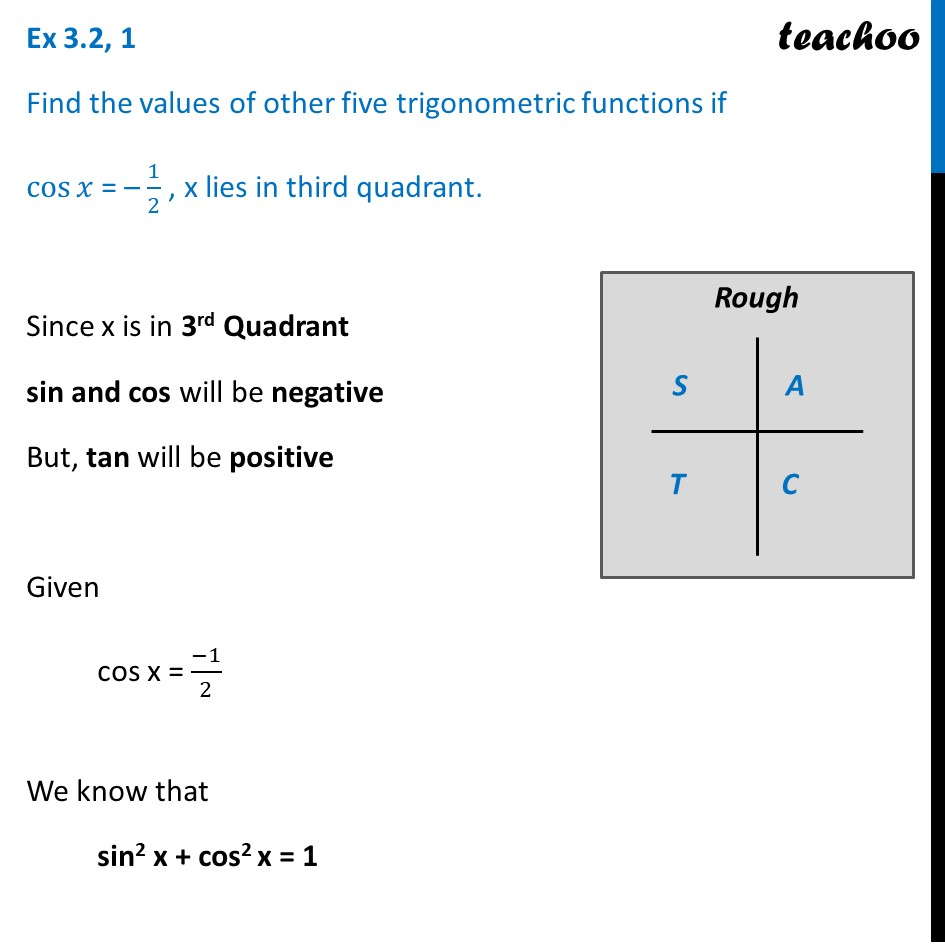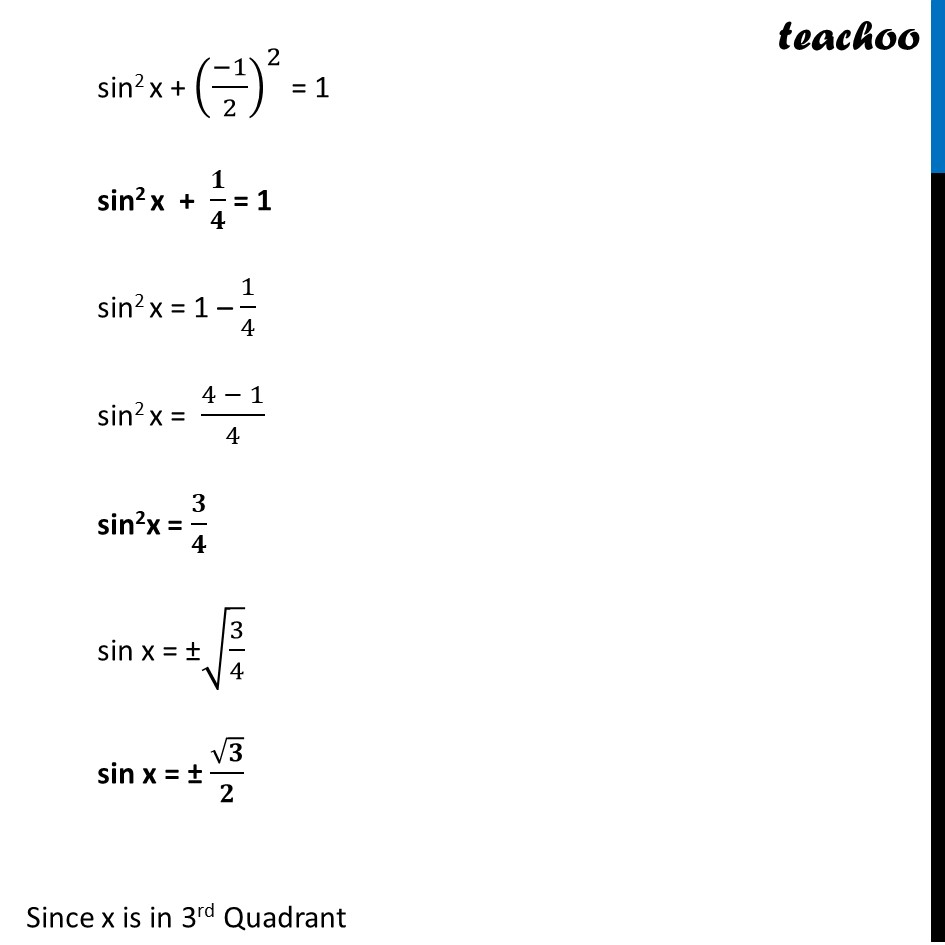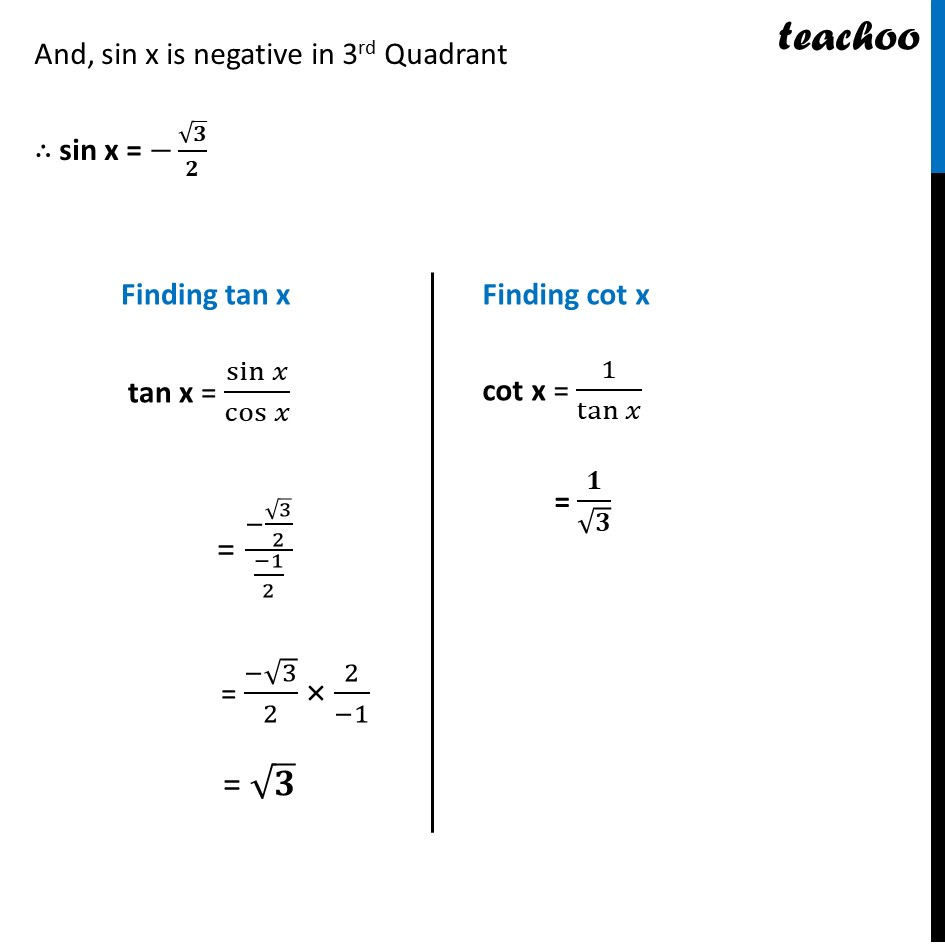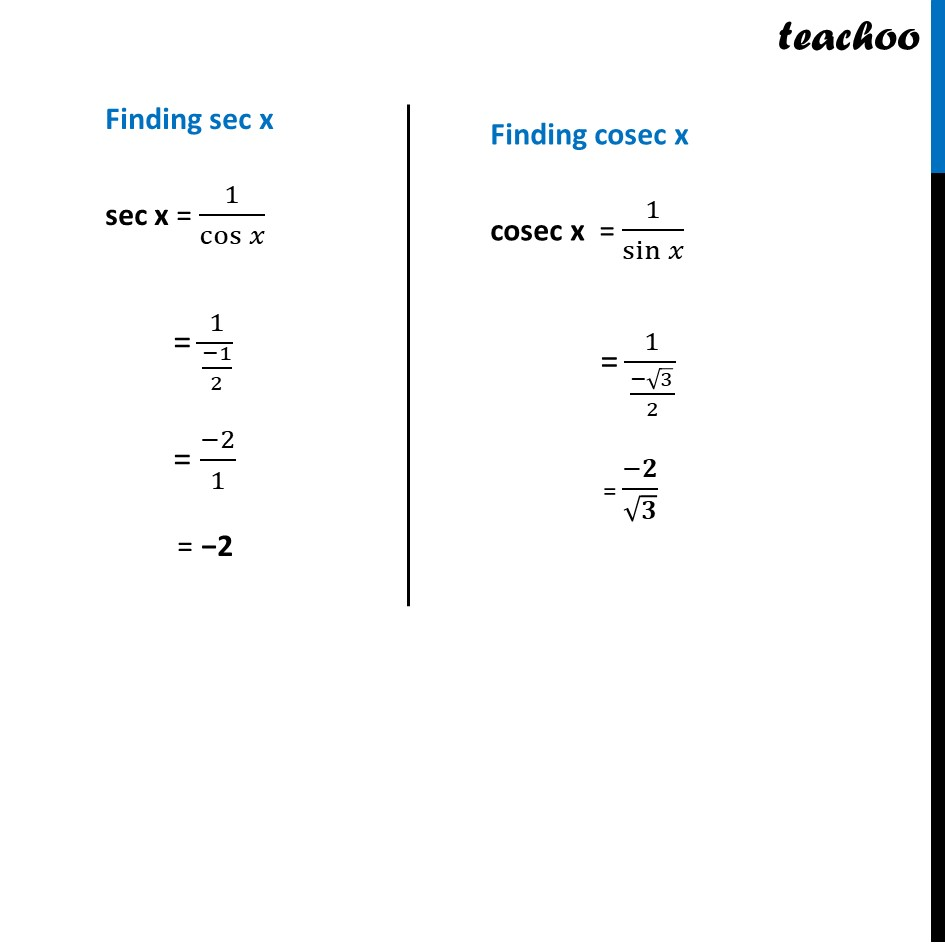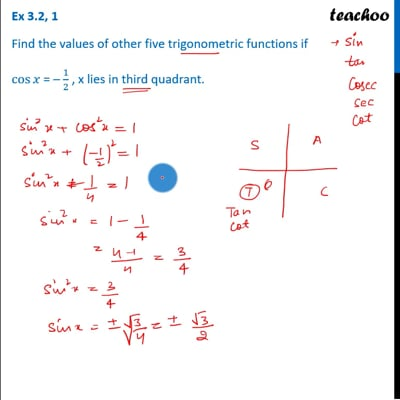This video is only available for Teachoo black users

Introducing your new favourite teacher - Teachoo Black, at only ₹83 per month

### Transcript

Ex 3.2, 1 Find the values of other five trigonometric functions if cos⁡𝑥 = – 1/2 , x lies in third quadrant. Since x is in 3rd Quadrant sin and cos will be negative But, tan will be positive Given cos x = (−1)/2 We know that sin2 x + cos2 x = 1 sin2 x + ((−1)/2)^2 = 1 sin2 x + 𝟏/𝟒 = 1 sin2 x = 1 – 1/4 sin2 x = (4 − 1)/4 sin2x = 𝟑/𝟒 sin x = ±√(3/4) sin x = ± √𝟑/𝟐 Since x is in 3rd Quadrant And, sin x is negative in 3rd Quadrant ∴ sin x = −√𝟑/𝟐 Finding tan x tan x = sin⁡𝑥/cos⁡𝑥 = (−√3/2)/((−1)/2) = (−√3)/2 × 2/(−1) = √𝟑 Finding cot x cot x = 1/tan⁡𝑥 = 𝟏/√𝟑 Finding sec x sec x = 1/cos⁡𝑥 = 1/((−1)/2) = (−2)/1 = −2 Finding cosec x cosec x = 1/sin⁡𝑥 = 1/((−√3)/2) = (−𝟐)/√𝟑